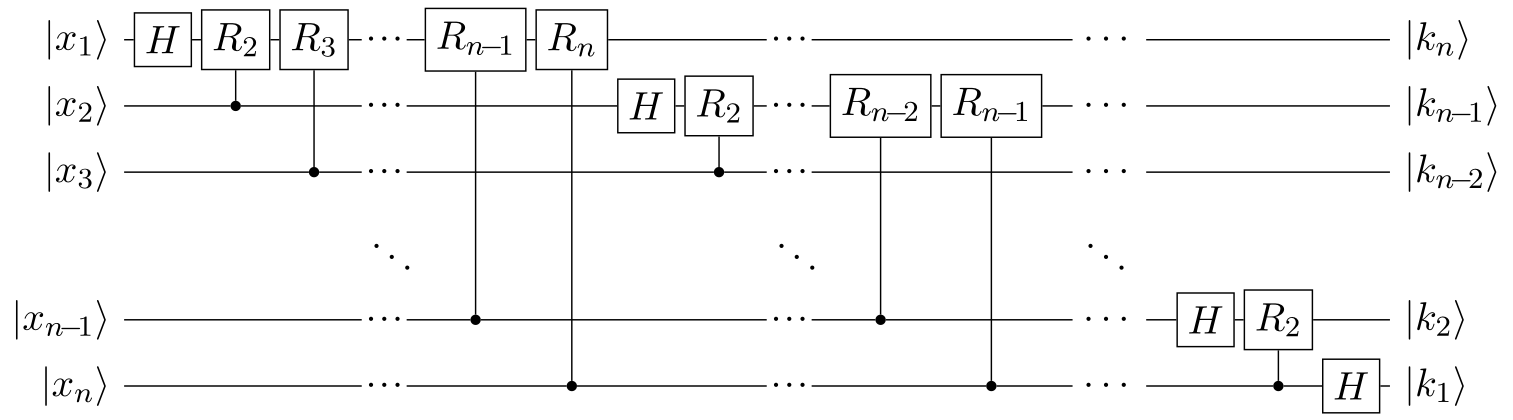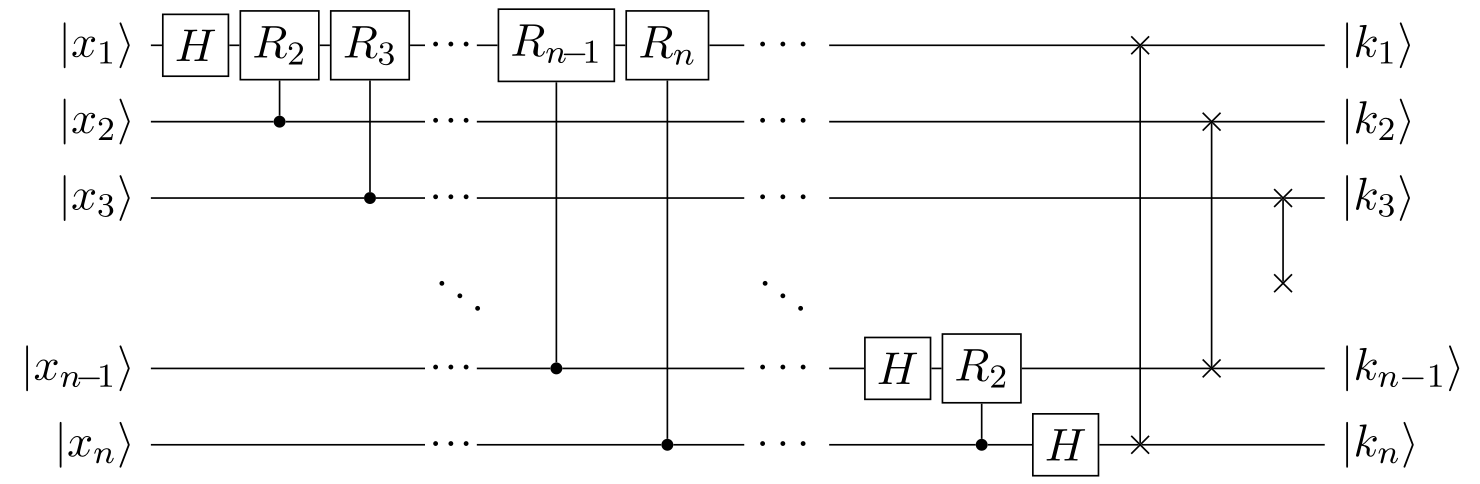Categories: Algorithms, Quantum information.

Quantum Fourier transform

The quantum Fourier transform (QFT) is a quantum counterpart of the classical discrete Fourier transform. It is defined like so, where $$\ket{x}$$ is an $$n$$-qubit computational basis state $$\ket{x_1} \cdots \ket{x_n}$$, and $$\omega_N$$ is an $$N$$th complex root of unity $$\omega_N^N = 1$$ with $$N = 2^n$$:

\begin{aligned} \boxed{ \ket{x} \:\to\: \frac{1}{\sqrt{N}} \sum_{k = 0}^{N - 1} \omega_N^{xk} \ket{k} } \end{aligned}

Note that $$\ket{x}$$ and $$\ket{k}$$ refer to the same basis set; we use these names to clarify which “space” we are considering. Furthermore, note the sign of the exponent of $$\omega_N$$, which is the opposite of the classical DFT convention. In other words, the forward QFT corresponds to the inverse DFT.

The inverse quantum Fourier transform (iQFT) has a different sign in the exponent:

\begin{aligned} \boxed{ \ket{k} \:\to\: \frac{1}{\sqrt{N}} \sum_{x = 0}^{N - 1} \omega_N^{-xk} \ket{x} } \end{aligned}

The above definitions of the QFT and iQFT describe the effect on a single basis vector $$\ket{x}$$, so the effect on an abitrary superposition follows from linearity, e.g. for the QFT:

\begin{aligned} \sum_{x = 0}^{N - 1} c_x \ket{x} \:\to\: \sum_{x = 0}^{N - 1} c_x \bigg( \frac{1}{\sqrt{N}} \sum_{k = 0}^{N - 1} \omega_N^{xk} \ket{k} \bigg) \end{aligned}

Classically, such a double sum takes $$\mathcal{O}(N^2) = \mathcal{O}(2^{2n})$$ time to evaluate naively, with a potential improvement to $$\mathcal{O}(N \log{N}) = \mathcal{O}(n 2^n)$$ for smarter algorithms. Quantum computers can do a QFT in $$\mathcal{O}(\log^2(N)) = \mathcal{O}(n^2)$$ time, and approximate it in $$\mathcal{O}(n \log{n})$$ time.

To find out how, we look at the forward QFT, which maps a basis state $$\ket{x}$$ to another state $$\ket{\tilde{x}}$$:

\begin{aligned} \ket{x} \:\to\: \ket{\tilde{x}} = \frac{1}{\sqrt{N}} \sum_{k = 0}^{N - 1} \omega_N^{xk} \ket{k} = \frac{1}{\sqrt{N}} \sum_{k = 0}^{N - 1} \exp\!\bigg( \frac{i 2 \pi x k}{N} \bigg) \ket{k} \end{aligned}

We can decompose $$k$$ into its binary representation $$k_1 2^{n-1} + k_2 2^{n-2} + ... + k_n 2^{0}$$:

\begin{aligned} \ket{\tilde{x}} &= \frac{1}{\sqrt{2^n}} \sum_{k = 0}^{2^n - 1} \exp\!\bigg( \frac{i 2 \pi x}{2^n} \sum_{j = 1}^{n} k_j 2^{n-j} \bigg) \ket{k} \\ &= \frac{1}{\sqrt{2^n}} \sum_{k = 0}^{2^n - 1} \exp\!\bigg( i 2 \pi x \sum_{j = 1}^{n} \frac{k_j}{2^j} \bigg) \ket{k} \end{aligned}

Expanding the exponential-of-a-sum into a product-of-exponentials then yields:

\begin{aligned} \ket{\tilde{x}} &= \frac{1}{\sqrt{2^n}} \sum_{k = 0}^{2^n - 1} \prod_{j = 1}^{n} \exp\!\bigg( i 2 \pi x \frac{k_j}{2^j} \bigg) \ket{k} \end{aligned}

If $$k_j = 0$$, the exponential is zero. We can thus separate this state into its individual qubits:

\begin{aligned} \ket{\tilde{x}} &= \bigotimes_{j = 1}^{n} \frac{1}{\sqrt{2}} \bigg( \ket{0} + \exp\!\Big( \frac{i 2 \pi x}{2^j} \Big) \ket{1} \bigg) \end{aligned}

Next, we use the trick from before: decompose $$x$$ as $$x_1 2^{n-1} + x_2 2^{n-2} + ... + x_n 2^{0}$$:

\begin{aligned} \ket{\tilde{x}} % &= \bigotimes_{j = 1}^{n} \frac{1}{\sqrt{2}} \bigg( \ket{0} + \exp\!\Big( \frac{i 2 \pi}{2^j} \sum_{r = 1}^{n} x_r 2^{r-1} \Big) \ket{1} \bigg) % \\ &= \bigotimes_{j = 1}^{n} \frac{1}{\sqrt{2}} \bigg( \ket{0} + \exp\!\Big( i 2 \pi \sum_{r = 1}^{n} x_r 2^{n-r-j} \Big) \ket{1} \bigg) \end{aligned}

The factor $$2^{n-r-j}$$ may be smaller or bigger than $$1$$, so it is convenient for us to use the following notation for non-integer binary numbers. Note the decimal point in the middle:

\begin{aligned} \left[ a_1 \cdots a_{d} \:.\: a_{d+1} \cdots a_{n} \right] = \sum_{r = 1}^{n} a_r 2^{d - r} \end{aligned}

In the above QFT state, the position of the decimal point is $$d = n - j$$, so we write it as:

\begin{aligned} \ket{\tilde{x}} &= \bigotimes_{j = 1}^{n} \frac{1}{\sqrt{2}} \bigg( \ket{0} + \exp\!\Big( i 2 \pi \big[ x_1 \cdots x_{n-j} \:.\: x_{n-j+1} \cdots x_n \big] \Big) \ket{1} \bigg) \end{aligned}

Because $$\exp(i 2 \pi m) = 1$$ for all integers $$m$$, we can discard bits before the decimal point:

\begin{aligned} \ket{\tilde{x}} &= \frac{1}{\sqrt{2^n}} \bigotimes_{j = 1}^{n} \bigg( \ket{0} + \exp\!\Big( i 2 \pi \big[ 0\:.\: x_{n-j+1} \cdots x_n \big] \Big) \ket{1} \bigg) \\ &= \frac{1}{\sqrt{2^n}} \bigg( \ket{0} + \exp\!\Big( i 2 \pi \big[ 0. x_n \big] \Big) \ket{1} \bigg) \otimes \bigg( \ket{0} + \exp\!\Big( i 2 \pi \big[ 0. x_{n-1} x_n \big] \Big) \ket{1} \bigg) \otimes \cdots \end{aligned}

Furthermore, each exponential can be factorized, with every factor containing one bit of $$x$$:

\begin{aligned} \exp\!\Big( i 2 \pi \big[ 0\:.\: x_{n-j+1} \cdots x_n \big] \Big) = \exp\!\Big( i 2 \pi \frac{x_{n-j+1}}{2} \Big) \cdots \exp\!\Big( i 2 \pi \frac{x_{n}}{2^{j}} \Big) \end{aligned}

This suggests a way to implement the QFT using quantum gates in a circuit. If the $$j$$th qubit is in $$\ket{+} = (\ket{0} + \ket{1})/\sqrt{2}$$, then for each bit $$x_{n-j+r}$$ where $$r \in \{1, ..., j\}$$:

• If $$x_{n-j+r} = 0$$, do nothing.
• If $$x_{n-j+r} = 1$$, add a relative phase $$2 \pi / 2^{r}$$.

The full QFT algorithm therefore proceeds as follows, for the $$(n\!-\!j\!+\!1)$$’th input $$\ket{x_{n-j+1}}$$:

1. Apply the Hadamard gate $$H$$. If $$x_{n-j+1} = 0$$, this puts the qubit in $$\ket{+}$$. If $$x_{n-j+1} = 1$$, this puts the qubit in $$\ket{+}$$, and then adds a phase $$\pi$$, yielding $$\ket{-}$$.
2. Apply the phase shift gate $$R_{\phi}$$ controlled by $$x_{n-j+2}$$, with angle $$\phi = 2 \pi / 2^{2}$$.
3. Apply $$R_{\phi}$$ controlled by $$x_{n-j+3}$$, with angle $$\phi = 2 \pi / 2^{3}$$
4. And so on, until the $$n$$th bit $$x_n$$ is reached, and used to control $$R_\phi$$ with $$\phi = 2 \pi / 2^{j}$$.

And so on, for each $$j \in \{1, ..., n\}$$, and we reach the above expression for $$\ket{\tilde{x}}$$. We started from the $$(n\!-\!j\!+\!1)$$’th input qubit, i.e. we read the input in reverse order. Therefore all the qubits need to be swapped back to front, either before or after the above algorithm is run.

The quantum circuit to execute the mentioned steps is illustrated below, excluding the swapping part to get the right order. Here, $$R_m$$ means $$R_\phi$$ with $$\phi = 2 \pi / 2^m$$:Again, note how the inputs $$\ket{x_j}$$ and outputs $$\ket{k_j}$$ are in the opposite order. The complete circuit, including the swapping at the end, therefore looks like this:For each of the $$n$$ qubits, $$\mathcal{O}(n)$$ gates are applied, so overall the QFT algorithm is $$\mathcal{O}(n^2)$$.

1. J.S. Neergaard-Nielsen, Quantum information: lectures notes, 2021, unpublished.
2. S. Aaronson, Introduction to quantum information science: lecture notes, 2018, unpublished.

© Marcus R.A. Newman, a.k.a. "Prefetch". Available under CC BY-SA 4.0.